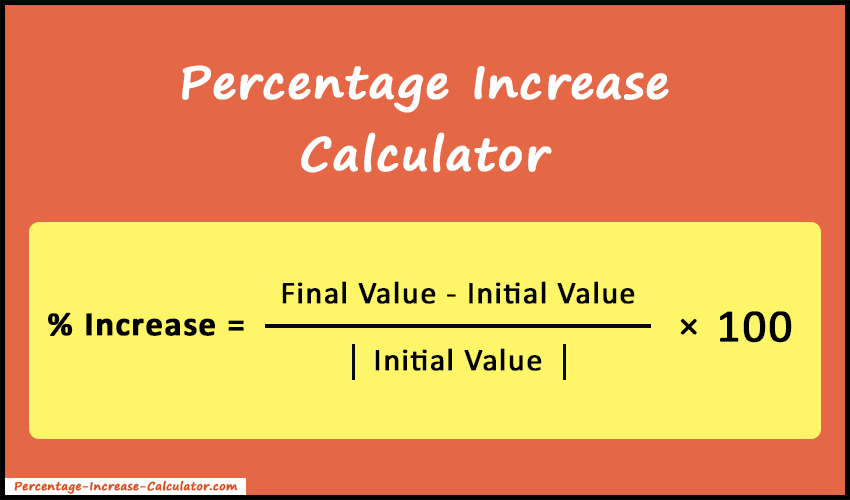# Percentage Increase Calculator

Percentage Increase Calculator takes an initial and final value and gives the percent(%) increase or decrease with calculation steps.

%
##### More Useful Tools
Percentage Change Calculator Mean Calculator Log Calculator

Percentage Increase Calculator helps you find out the increase or decrease from one value to another in terms of percentage. Also, it's a free-to-use online tool and it makes your calculations much easier, faster, and more accurate. Just enter the initial and final values and press the Calculate button. As a result, it gives an increase or decrease in terms of percentage. Also, it shows the step-by-step calculation results with the percentage increase formula.#### What is Percentage Increase?

The percentage is used in many settings in order to compare two values. We often have to compare the values of a single variable at two different points or times. Both percent increase and decrease are measures of change in percent, that is the extent to which variable gains or loses intensity, magnitude, extent, or value. Lastly, we get the results by comparing the initial and final values according to a formula.

The term percentage increase is also called percentage change. It refers to the difference between the final and initial values of a quantity. If the percentage increase is a negative value, then we can say that there is a percentage decrease in quantity.

Also, it is very useful in analyzing how the value has changed over time. It is quite similar to an absolute increase. However, the former is more used when comparing multiple data sets.

For example, consider two changes, the first one is from 1 to 51 and another from 50 to 100. Both changes have an absolute change of 50. But the percentage change in the first case is 5000%, while in the second case, it is 100%. Thus, we can say that the first change grew a lot more. Moreover, the percentage increase is the most common way of measuring growth.

### How to Calculate Percentage Increase?

The simplest and easiest possible way to calculate percentage increase is to use our percentage increase calculator. Along with that if you wish to learn the standard mathematical procedure to calculate the percentage increase on pen and paper, then follow the method below.

Firstly, let's know the Percentage Increase Formula.

#### Percentage Increase Formula

% Increase =
 Final - Initial | Initial |
×  100

Now follow the steps below to calculate the percent increase.

1. Identify the initial or starting value and the final value.
2. Enter the values into the formula.
3. Subtract the initial value from the final value and then divide it by the initial value.
4. Lastly, multiply the value obtained in the previous step by 100.
5. Finally, the value you get is a percent increase or decrease.
##### Example 1:

Consider you invest \$1000 which is increased to \$1500 in a period of one year. Then, as per the method discussed above, the percentage increase of your investment will be:

Solution:

((1500 – 1000) / 1000) × 100
(500 / 1000) × 100 = 50% (increase)

Let's take one more example of a percentage decrease.

##### Example 2:

Suppose you invested \$1500, whose value after one year decreased to \$800, then the percent decrease would be:

Solution:

((800 – 1500) / 1500) × 100
(-700 / 1500) × 100 = -46.67% (decrease)

### How to use Percentage Increase Calculator?

Follow the instructions below to use the calculator.

1. Firstly, open the Percentage Increase Calculator on any device with an active internet connection and browser support.
2. Next, enter the initial and final values in the respective fields.
3. Lastly, press the Calculate button.
4. The final results will be instantly displayed on your device's screen with calculation steps.
5. Press the Reset button to clear all the input as well as output fields.

#### Features of Percentage Increase Calculator

• Simple and easy to use: The interactive and responsive user interface of our tool makes it easy for all to understand and use.
• All in one tool: It's a comprehensive tool that is used to find the percentage increase as well as decrease. In short, it's a one-stop destination for all users.
• Fast and accurate results: It gives accurate results too in a few seconds.
• Free to use: It's totally free to use for all users. Also, there are no calculation limits or hidden charges.
• Device Compatibility: It can be used on any type of device, be it a smartphone, tablet, laptop, or personal computer. The tool can also run on different operating systems and browsers.
• Security: You don't have to worry about your data getting stolen or leaked. Because no data is stored or sent to the server. So, our tool is completely safe to use.

### FAQs

% Increase = (Final Number - Starting Number / Starting Number) × 100

1. Subtract the starting value by the final value and then divide it by starting value.
2. Lastly, multiply the value that you get from the above step by 100 to get the percentage increase.
3. If you get the negative value, it means there is a decrease in percentage.

Yes, our tool gives you the increase and decrease of both results.

No, you can calculate as many times as you wish.

Our tool is simple and easy to use. Also, we don't charge a single penny from you to use it. Moreover, it gives fast and accurate results. This tool lets you easily calculate percentage change and eliminates the difficulty you might face while solving it on pen and paper.